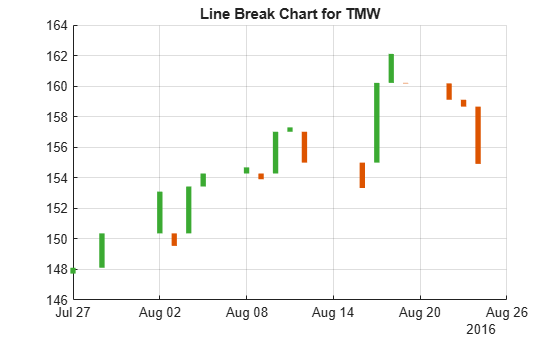# linebreak

Line break chart

`linebreak` is updated to accept data input as a matrix, `timetable`, or `table`.

The syntax for `linebreak` has changed. Previously, when using table input, the first column of dates could be serial date numbers, date character vectors, or datetime arrays, and you were required to have specific number of columns.

When using table input, the new syntax for `linebreak` supports:

• No need for time information. If you want to pass in date information, use timetable input.

• No requirement of specific number of columns. However, you must provide valid column names. `linebreak` must contain a column named `‘price’` (case insensitive).

## Syntax

``linebreak(Data)``
``h = linebreak(ax,Data)``

## Description

example

````linebreak(Data)` plots the asset data, in a line break chart.```

example

````h = linebreak(ax,Data)` adds an optional argument for `ax`. ```

## Examples

collapse all

Load the file `SimulatedStock.mat`, which provides a timetable (`TMW`) for financial data for TMW stock. This Linebreak chart is for closing prices of the stock TMW for the most recent 21 days. Note that the variable name of asset price is be renamed to `'Price'` (case insensitive).

```load SimulatedStock.mat TMW.Properties.VariableNames{'Close'} = 'Price'; linebreak(TMW(end-20:end,:)) title('Line Break Chart for TMW')```## Input Arguments

collapse all

Data for a series of prices, specified as a matrix, table, or timetable. Timetables and tables with `M` rows must contain a variable named `'Price'` (case insensitive).

Data Types: `double` | `table` | `timetable`

(Optional) Valid axis object, specified as an axes object. The linebreak plot is created in the axes specified by `ax` instead of in the current axes (`ax = gca`). The option `ax` can precede any of the input argument combinations.

Data Types: `object`

## Output Arguments

collapse all

Graphic handle of the figure, returned as a handle object.

## Version History

Introduced in R2008a